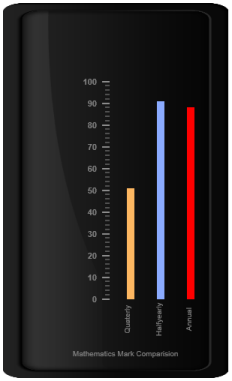# Bar Pointers

21 Apr 20175 minutes to read

Bar Pointer value points out the actual value set in the Linear Gauge as marker pointer. You can set the values of the various bar pointer attributes such as value, width, border and color in bar pointer collection. You can also customize the pointers to improve the appearance of gauge.

You can add Bar Pointer collection directly to the scale object. Refer the following code example.

• CSHTML
• ``````<ej-linear-gauge id="LinearGauge" enable-animation="false" value="78" >
<e-frame  inner-width="8" outer-width="10"
background-image-url="../images/gauge/Gauge_linear_light.png" ></e-frame>
<e-linear-scale-collections>
<e-linear-scales width="0" show-bar-pointers="true" show-marker-pointers="false"
type="@ScaleType.RoundedRectangle" >
<e-border color="transparent" width="0"></e-border>
<e-bar-pointer-collections>
<e-bar-pointers width="5" bar-pointerdistance-from-scale="15"
bar-pointer-background-color="Grey">
</e-bar-pointers>
</e-bar-pointer-collections>
<e-linear-tick-collections>
<e-linear-ticks type="@TickType.MajorInterval"  width="2" color="#8c8c8c" >
<e-distance-from-scale x="7" y="0"></e-distance-from-scale>
</e-linear-ticks>
<e-linear-ticks type="@TickType.MinorInterval" height="6"  width="1" color="#8c8c8c" >
<e-distance-from-scale x="7" y="0"></e-distance-from-scale>
</e-linear-ticks>
</e-linear-tick-collections>
</e-linear-scales>
</e-linear-scale-collections>
</ej-linear-gauge>``````

Execute the above code to render the following output.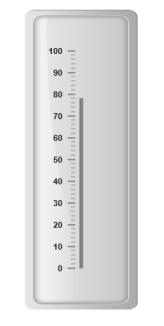Linear Gauge with bar pointer

Bar pointer value is also important element in the Linear Gauge as it indicates the gauge value. Real purpose of the Linear Gauge is based on the pointer value. You can set the bar pointer value either directly during rendering the control or it can be achieved by public method.

• CSHTML
• ``````<ej-linear-gauge id="LinearGauge" enable-animation="false"  >
<e-frame  inner-width="8" outer-width="10"
background-image-url="../images/gauge/Gauge_linear_light.png" ></e-frame>
<e-linear-scale-collections>
<e-linear-scales width="0" show-bar-pointers="true" show-marker-pointers="false"
type="@ScaleType.RoundedRectangle" >
<e-border color="transparent" width="0"></e-border>
<e-bar-pointer-collections>
<e-bar-pointers width="5" bar-pointerdistance-from-scale="15" bar-pointer-value="91"
bar-pointer-background-color="Grey">
</e-bar-pointers>
</e-bar-pointer-collections>
<e-linear-tick-collections>
<e-linear-ticks type="MajorInterval"  width="2" color="#8c8c8c" >
<e-distance-from-scale x="7" y="0"></e-distance-from-scale>
</e-linear-ticks>
<e-linear-ticks type="MinorInterval" height="6"  width="1" color="#8c8c8c" >
<e-distance-from-scale x="7" y="0"></e-distance-from-scale>
</e-linear-ticks>
</e-linear-tick-collections>
</e-linear-scales>
</e-linear-scale-collections>
</ej-linear-gauge>``````

Execute the above code to render the following output.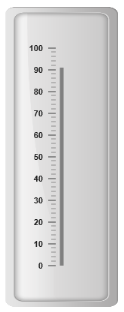## Pointer Styles

### Appearance

• Based on the value, the bar pointer points out the label value. You can set the bar pointer width using width property and you can also adjust the opacity of the pointer using opacity property that holds the value between 0 and 1. You can add the gradient effects to the pointer using gradient object.
• The marker pointer border is modified with the object border. It has two border property, color and width which are used to customize the border color of the scale and border width of the marker pointer. The background color can be customized with attribute backgroundColor.
• CSHTML
• ``````<ej-linear-gauge id="LinearGauge" enable-animation="false"  >
<e-frame  inner-width="8" outer-width="10"
background-image-url="../images/gauge/Gauge_linear_light.png" ></e-frame>
<e-linear-scale-collections>
<e-linear-scales width="0" show-bar-pointers="true" show-marker-pointers="false"
type="@ScaleType.RoundedRectangle" >
<e-border color="transparent" width="0"></e-border>
<e-bar-pointer-collections>
<e-bar-pointers width="10" bar-pointerdistance-from-scale="15" bar-pointer-value="91"
bar-pointer-background-color="Red" bar-pointer-opacity="0.7">
</e-bar-pointers>
</e-bar-pointer-collections>
<e-linear-tick-collections>
<e-linear-ticks type="MajorInterval"  width="2" color="#8c8c8c" >
<e-distance-from-scale x="7" y="0"></e-distance-from-scale>
</e-linear-ticks>
<e-linear-ticks type="MinorInterval" height="6"  width="1" color="#8c8c8c" >
<e-distance-from-scale x="7" y="0"></e-distance-from-scale>
</e-linear-ticks>
</e-linear-tick-collections>
</e-linear-scales>
</e-linear-scale-collections>
</ej-linear-gauge>``````

Execute the above code to render the following output.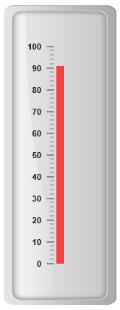## Positioning the pointer

• Bar pointer can be positioned with two properties such as distanceFromScale and placement. The distanceFromScale property defines the distance between the scale and pointer element.
• The placement property is used to locate the pointer with respect to scale either inside or outside the scale or along the scale. It is an enumerable data type.
• CSHTML
• ``````<ej-linear-gauge id="LinearGauge" enable-animation="false"  >
<e-frame  inner-width="8" outer-width="10"
background-image-url="../images/gauge/Gauge_linear_light.png" ></e-frame>
<e-linear-scale-collections>
<e-linear-scales width="0" show-bar-pointers="true" show-marker-pointers="false"
type="@ScaleType.RoundedRectangle" >
<e-border color="transparent" width="0"></e-border>
<e-bar-pointer-collections>
<e-bar-pointers width="10" bar-pointerdistance-from-scale="40" bar-pointer-value="91"
bar-pointer-background-color="#8BABFF" >
</e-bar-pointers>
</e-bar-pointer-collections>
<e-linear-tick-collections>
<e-linear-ticks type="MajorInterval"  width="2" color="#8c8c8c" >
<e-distance-from-scale x="7" y="0"></e-distance-from-scale>
</e-linear-ticks>
<e-linear-ticks type="MinorInterval" height="6"  width="1" color="#8c8c8c" >
<e-distance-from-scale x="7" y="0"></e-distance-from-scale>
</e-linear-ticks>
</e-linear-tick-collections>
</e-linear-scales>
</e-linear-scale-collections>
</ej-linear-gauge>``````

Execute the above code to render the following output.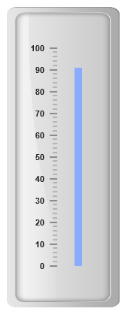## Multiple Bar Pointers

Linear Gauge can contain multiple bar pointers on it. You can use any combination and any number of pointers in a gauge. That is, a Gauge can contain any number of marker pointer and any number of bar pointers. Refer the following code example containing multiple bar pointers.

• CSHTML
• ``````<ej-linear-gauge id="LinearGauge" enable-animation="false"height="500" width="300" >
<e-frame  inner-width="8" outer-width="10"
background-image-url="../images/gauge/Gauge_linear_dark1.png" ></e-frame>
<e-linear-scale-collections>
<e-linear-scales width="0" show-bar-pointers="true" show-marker-pointers="false"
show-custom-labels="true">
<e-border color="transparent" width="0"></e-border>
<e-bar-pointer-collections>
<e-bar-pointers width="10" bar-pointerdistance-from-scale="60" bar-pointer-value="91"
bar-pointer-background-color="#8BABFF" >
</e-bar-pointers>
<e-bar-pointers width="10" bar-pointerdistance-from-scale="20" bar-pointer-value="51"
bar-pointer-background-color="#FDB761" >
</e-bar-pointers>
<e-bar-pointers width="10" bar-pointerdistance-from-scale="100" bar-pointer-value="88"
bar-pointer-background-color="Red" >
</e-bar-pointers>
</e-bar-pointer-collections>
<e-linear-tick-collections>
<e-linear-ticks type="MajorInterval"  width="2" color="#8c8c8c" >
<e-distance-from-scale x="7" y="0"></e-distance-from-scale>
</e-linear-ticks>
<e-linear-ticks type="MinorInterval" height="6"  width="1" color="#8c8c8c" >
<e-distance-from-scale x="7" y="0"></e-distance-from-scale>
</e-linear-ticks>
</e-linear-tick-collections>
<e-custom-label-collections>
<e-custom-labels value="Mathematics Mark Comparision">
<e-linear-position x="55" y="97"></e-linear-position>
</e-custom-labels>
<e-custom-labels value="Halfyearly" text-angle="90" >
<e-linear-position x="72" y="87"></e-linear-position>
</e-custom-labels>
<e-custom-labels value="Quarterly" text-angle="90" >
<e-linear-position x="56" y="87"></e-linear-position>
</e-custom-labels>
<e-custom-labels value="Annual" text-angle="90">
<e-linear-position x="87" y="87"></e-linear-position>
</e-custom-labels>
</e-custom-label-collections>
</e-linear-scales>
</e-linear-scale-collections>
</ej-linear-gauge>``````

Execute the above code to render the following output.